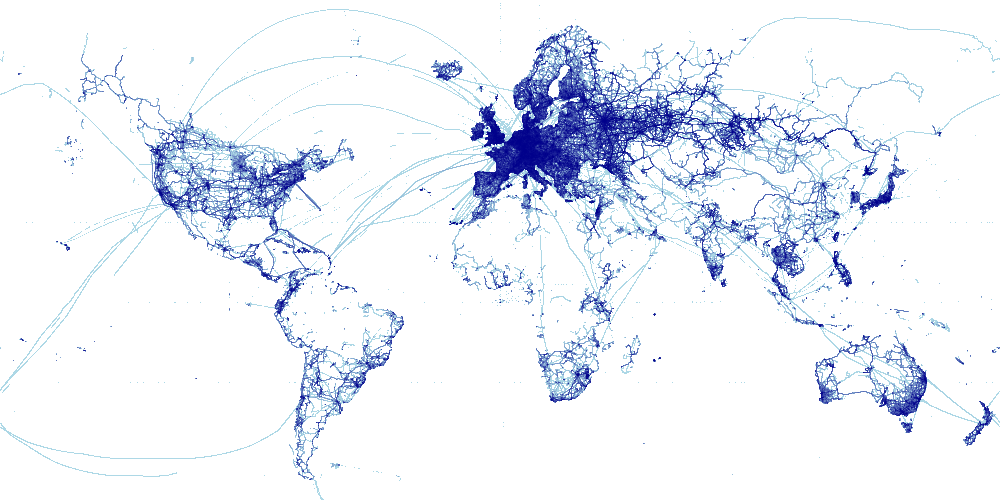# Osm-3billion¶

## Datashading a 2.7-billion-point Open Street Map database¶

Most datashader examples use "medium-sized" datasets, because they need to be small enough to be distributed over the internet without racking up huge bandwidth charges for the project maintainers. Even though these datasets can be relatively large (such as the 1-billion point Open Street Map example), they still fit into memory on a 16GB laptop.

Because Datashader supports Dask dataframes, it also works well with truly large datasets, much bigger than will fit in any one machine's physical memory. On a single machine, Dask will automatically and efficiently page in the data as needed, and you can also easily distribute the data and computation across multiple machines. Here we illustrate how to work "out of core" on a single machine using a 22GB OSM dataset containing 2.7 billion points.

The data is taken from Open Street Map's (OSM) bulk GPS point data, and is unfortunately too large to distribute with Datashader (8.4GB compressed). The data was collected by OSM contributors' GPS devices, and was provided as a CSV file of latitude,longitude coordinates. The data was downloaded from their website, extracted, converted to use positions in Web Mercator format using datashader.utils.lnglat_to_meters(), sorted using spacial indexing, and then stored in a parquet file for fast partition-based access. To run this notebook, you would need to do the same process yourself to obtain osm-3billion.parq. Once you have it, you can follow the steps below to load and plot the data.

In :
import datashader as ds


In :
%%time

CPU times: user 1.35 s, sys: 20.9 ms, total: 1.37 s
Wall time: 1.38 s

In :
df.head()

Out:
x y
0 -17219646.0 -19219556.0
1 -17382392.0 -18914976.0
2 -16274360.0 -17538778.0
3 -17219646.0 -16627029.0
4 -16408889.0 -16618700.0

### Aggregation¶

First, we define some spatial regions of interest and create a canvas to provide pixel-shaped bins in which points can be aggregated:

In :
def bounds(x_range, y_range):
x,y = lnglat_to_meters(x_range, y_range)
return dict(x_range=x, y_range=y)

Earth       = ((-180.00, 180.00), (-59.00, 74.00))
France      = (( -12.00,  16.00), ( 41.26, 51.27))
Paris       = ((   2.05,   2.65), ( 48.76, 48.97))

plot_width = 1000
plot_height = int(plot_width*0.5)

cvs = ds.Canvas(plot_width=plot_width, plot_height=plot_height, **bounds(*Earth))


Next we aggregate the data to produce a fixed-size aggregate array. This process may take up to a minute, so we provide a progress bar using dask:

In :
with diag.ProgressBar(), diag.Profiler() as prof, diag.ResourceProfiler(0.5) as rprof:
agg = cvs.points(df, 'x', 'y')

[########################################] | 100% Completed |  0.3s
[########################################] | 100% Completed | 30.8s


We can now visualize this data very quickly, ignoring low-count noise as described in the 1-billion point OSM version:

In :
tf.shade(agg.where(agg > 15), cmap=["lightblue", "darkblue"])

Out:Looking at smaller areas is much quicker, thanks to SpatialPointsFrame:

In :
def plot(x_range, y_range):
cvs = ds.Canvas(plot_width=plot_width, plot_height=plot_height, **bounds(x_range, y_range))
agg = cvs.points(df, 'x', 'y')

%time plot(*France)

CPU times: user 39.1 s, sys: 12.5 s, total: 51.7 s